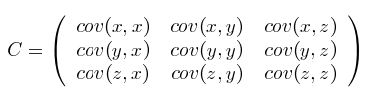0%

• 均值：$\bar{X}=\frac{\sum_{i=1}^n X_{i}}{n}$
• 标准差：$s=\sqrt{\frac{\sum_{i=1}^n (X_{i}-\bar{X})^2}{n-1}}$
• 方差：$s^2=\frac{\sum_{i=1}^n (X_{i}-\bar{X})^2}{n-1}$

$var(X)=\frac{\sum_{i=1}^n (X_{i}-\bar{X})(X_{i}-\bar{X})}{n-1}$

$cov(X,Y)=cov(Y,X)=var(X)=\frac{\sum_{i=1}^n (X_{i}-\bar{X})(Y_{i}-\bar{Y})}{n-1}$

$C_{n\times n}=(c_{i,j},c_{i,j}=cov(Dim_{i},Dim_{j}))$Buy Me A Coffee
Title - Artist
0:00